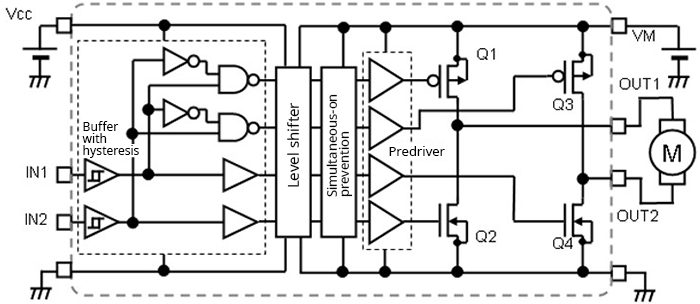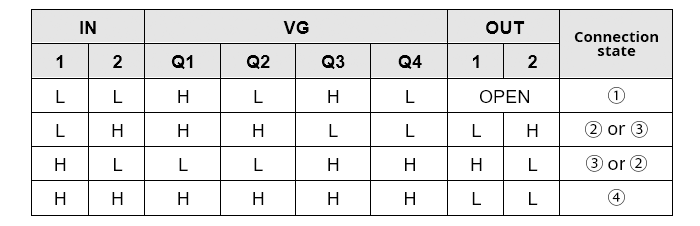Technical Information Site of Power Supply Design

2022.06.22 motor

# Driving Brushed DC Motors with an H-Bridge: Switching Output States

Brushed DC Motor

In the previous article, the principles of H-bridge circuits were explained. Here, control (switching) of an H-bridge is explained.

## H-bridge Circuit: Switching Output States

In the previous article it was explained that there are the following four possible combinations of connections when applying a voltage to the two terminals of a motor.

① Neither of the two terminals are connected to anything.
② One terminal is connected to the positive (+) terminal of a DC power supply,
the other is connected to the negative (-) terminal.
③ The motor is connected to the DC power supply with the polarity opposite that of ②.
④ The two terminals are connected to each other.

These four states can be created using combinations of two two-valued inputs. As a simple example, if there are two logic input terminals, 2x2=4 combinations are obtained. Below, in an example of a driver with an integrated H-bridge circuit for a brushed DC motor, schematic internal function blocks are indicated.The logic circuit to switch the H-bridge states is the block enclosed by a dashed line with IN1 and IN2 as inputs. The following is the truth value table for this logic circuit.VG states for input logic values IN are shown. The VG values should be understood to be the gate voltage states of the MOSFETs Q1 to Q4. For example, a VG value for Q1 of H means that an H level voltage is being applied; it should be noted that this does not directly indicate that Q1 is turned on or turned off.

This H-bridge uses a combination of P-channel MOSFETs (Q1, Q3) and N-channel MOSFETs. The MOSFETs are paired with P-channel MOSFETs on the high side and N-channel MOSFETs on the low side (Q1 and Q2, Q3 and Q4); a drain and source on each side are connected to the outputs OUT1 (the pair Q1 and Q2) and OUT2 (the pair Q3 and Q4).

As the reader will notice on viewing the block diagram, active L labels appear at the gates of the P-channel MOSFETs Q1 and Q3, so that the MOSFETs turn on when VG is L. Q2 and Q4 are active H, and so turn on when VG is H. Judging from this alone, the circuit is the same as a CMOS inverter logic circuit; but the gates are controlled separately by IN1 and IN2.

The OUT states correspond to the four required states that are created. OPEN means that all the MOSFETs are turned off in a high-impedance state. L means connection to GND via the MOSFET, and H similarly means connection to the motor power supply VM.

The level shifter and simultaneous-on prevention function shown in the function block diagram are not directly related to the H-bridge control logic, but are examples of functions that are necessary for hardware control.

The level shifter is a circuit that converts the output voltage of the low-voltage logic circuit so as to be compatible with high-voltage MOSFET driving. The logic circuit is generally designed to operate at 3.3 V or 2.5 V logic levels, but H-bridges operate at the higher voltages (5, 12, or 24 V, or the like) needed for motor driving. Hence the low-voltage logic output cannot be used as-is for driving, and so a level shifting circuit is necessary.

The simultaneous-on prevention circuit functions to prevent instantaneous overlap of the on-states of both high-side transistors and low-side transistors during on-off switching. Such simultaneous-on phenomena are a result of minute timing problems with switching in the logic. A well-known example in synchronous rectification switching power supplies is called shoot-through; during the instant that the on-states overlap, a shoot-through current flows from the power supply to GND, threatening to culminate in failure of the switching transistors or the IC.

While the discussion has digressed somewhat, the main point is that a logic input of two bits is used to obtain the four states of an H-bridge circuit.

#### Key Points:

・Switching between the four connection states of an H-bridge circuit is possible using two-bit logic.

・Many actual brushed DC motor driver ICs include, in addition to the H-bridge and a control logic circuit, a level shifter and a simultaneous-on prevention function.